# Square

Calculate area of the square with diagonal 64 cm.

Result

S =  2048 cm2

#### Solution:Leave us a comment of example and its solution (i.e. if it is still somewhat unclear...):Be the first to comment!#### To solve this verbal math problem are needed these knowledge from mathematics:

Pythagorean theorem is the base for the right triangle calculator.

## Next similar examples:

1. SquareCalculate the perimeter and the area of square with a diagonal length 30 cm.
2. Areaf of ST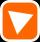It is given square DBLK with side |BL|=13. Calculate area of triangle DKU if vertex U lie on line LB.
3. HypotenuseCalculate the length of the hypotenuse of a right triangle if the length of one leg is 4 cm and its content area is 16 square centimeters.
4. The cellar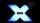Mr Novák has a cellar and a cellar window in the chalet has 0.6 meter square window. The window wishes to place an X-shaped grid in a square. He uses iron welded bars. Calculate the lengths of individual bars and what the total length of the bars he has t
5. Diagonal of squareCalculate the side of a square when its diagonal is 10 cm.
6. Octagonal mat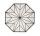Octagonal mat formed from a square plate with a side of 40 cm so that every corner cut the isosceles triangle with leg 3.6 cm. What is the content area of one mat?
7. Dog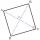Dog is tied to a chain, which is mounted in a corner of the yard. Yard has the shape of a square with a side length of 20 meters. The same long is also dogchain. Are there places in the yard where dog can't reach?
8. Square2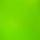Side of the square is a = 6.2 cm, how long is its diagonal?
9. Trio 2Decide whether trio of numbers is the side of a right triangle: 26,24,10.
10. RT 10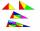Area of right triangle is 84 cm2 and one of its cathethus is a=10 cm. Calculate perimeter of the triangle ABC.
11. Right triangle ABCCalculate the perimeter and area of a right triangle ABC, if you know the length of legs 4 cm 5.5 cm and 6.8 cm is hypotenuse.
12. Median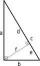In the right triangle are sides a=41 dm b=42 dm. Calculate the length of the medians tc to the hypotenuse.
13. Four ropesTV transmitter is anchored at a height of 44 meters by four ropes. Each rope is attached at a distance of 55 meters from the heel of the TV transmitter. Calculate how many meters of rope were used in the construction of the transmitter. At each attachment.
14. Broken treeThe tree is broken at 4 meters above the ground and the top of the tree touches the ground at a distance of 5 from the trunk. Calculate the original height of the tree.
15. SatinSanusha buys a piece of satin 2.4 m wide. The diagonal length of the fabric is 4m. What is the length of the piece of satin?
16. WindbreakA tree at a height of 3 meters broke in the windbreak. Its peak fell 4.5 m from the tree. How tall was the tree?
17. Broken treeThe tree was 35 meters high. The tree broke at a height of 10 m above the ground. Top but does not fall off it refuted on the ground. How far from the base of the tree lay its peak?